# SSC Ratio and Proportion Questions with Answers

#### Ratio and Proportion

An excellent collection of SSC Ratio and proportion questions and answers with detailed explanations for competitive exams. Given below are some of the most repeated practice questions on Ratio and proportion for SSC CGL, CHSL, JE, GD constable, Stenographer, MTS, and CPO exams. Go through this very important online quiz based on model and previous year asked questions from SSC Ratio and proportion with solutions to ace the exam.

### Take a free SSC CHSL Mock

Thousands of students have taken Cracku's Free SSC CHSL Mock.

Instructions

For the following questions answer them individually

Question 1

A rational number between 3/4 and 3/8 is

Question 2

The ratio of the income to the expenditure of a family is 10:7. If the family's expenses are Rs. 10,500, then savings of the family is

Question 3

The diameters of two circles are the side of a square and the diagonal of the square. The ratio of the areas of the smaller circle and the larger circle is

Question 4

Two circles with radii 5cm and 8 cm touch each other externally at a point A. If a straight line through the point A cuts the circles at points P and Q respectively, the AP : AQ is

Question 5

Monthly incomes of A and B are in the ratio of 4 : 3 and their expenses bear the ratio 3 : 2. Each of them saves Rs. 6,000 at the end of the month, then the monthly income of A is

Question 6

The ratio between two numbers is 2 : 3. If each number is increased by 4, the ratio between them becomes 5 : 7. The difference between the numbers is

Question 7

The ratio of the quantities on an acid and water in a mixture is 1 : 3. If 5 liters of acid is further added to the mixture, the new ratio becomes 1 : 2. The quantity of new mixture in litres is

Question 8

$$\frac{2+\sqrt{3}}{2-\sqrt{3}}+\frac{2-\sqrt{3}}{2+\sqrt{3}}+\frac{\sqrt{3}+1}{\sqrt{3}-1}$$ =

Question 9

If the ratio of cost price and selling price of an article be as 10:11, the percentage of profit is

Question 10

The sum of the series (1 + 0.6 + 0.06 + 0.006 + 0.0006 + ....) is

Instructions

Directions :The pie chart, given here, represents the number of valid votes obtained by four students who contested election for school leadership. The total number of valid votes polled was 720. Observe the chart and answer the questions based on it.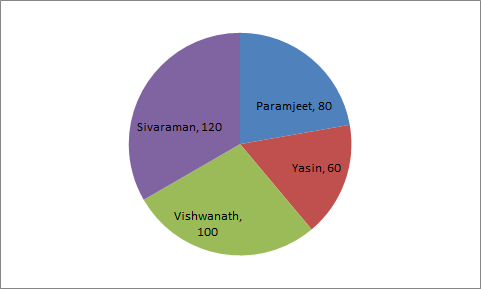Number represented in pie-chart are in degrees.

Question 11

By how many votes did the winner defeat his nearest rival ?

Instructions

Directions:The pie chart given below show the spending of a family on various heads during a month. Study the graph and answer the questions.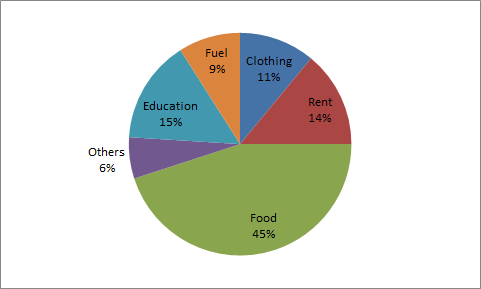Question 12

What is the ratio of the expenses of Education to the expenses on Food?

Instructions

For the following questions answer them individually

Question 13

$$\frac{4\sqrt{3} + 5\sqrt{2}}{\sqrt{48} + \sqrt{18}} = a + b\sqrt{6}$$

Question 14

The sides of a triangle are in the ration 2 : 3 : 4. The perimeter of the triangle is 18 cm. The area (in cm2) of the triangle is

Question 15

Shri X goes to his office by scooter at a speed of 30 km/h and reaches 6 minutes earlier. If he goes at a speed of 24 km/h, he reaches 5 minutes late. The distance of his office is

Question 16

A certain distance is covered at a certain speed. If half of this distance is covered in double the time, the ratio of the two speeds is

Question 17

If x : y = 5 : 6, then (3x - 2y ) (y - x ) is

Question 18

If a : b = 2 : 3, b :c = 4 : 5 and c : d=6:7,then a : d=

Instructions

Study the following Histogram and answer the following questions.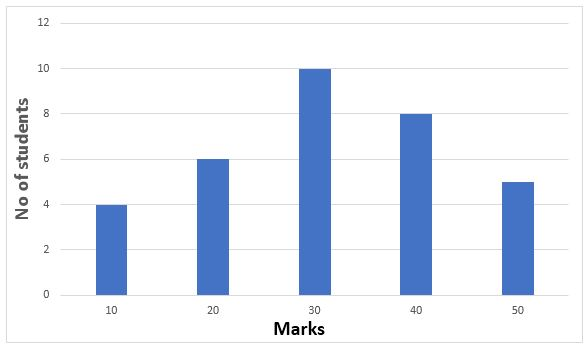Question 19

The ratio of the students obtaining marks in the first and last interval is

Instructions

For the following questions answer them individually

Question 20

Lokesh starts a business with Rs. 240000. After 3 months Vishal joins him with Rs 210000. At the end of the year what will be the ratio of their profit?

Instructions

Directions :The pie chart, given here, represents the number of valid votes obtained by four students who contested election for school leadership. The total number of valid votes polled was 720. Observe the chart and answer the questions based on it.Number represented in pie-chart are in degrees.

Question 21

What was the minimum number of votes obtained by any candidate ?

Instructions

For the following questions answer them individually

Question 22

The simplest value of
$$\frac{1}{\sqrt{2} + \sqrt{3}} + \frac{1}{\sqrt{3} + \sqrt{4}} + \frac{1}{\sqrt{4} + \sqrt{5}} + \frac{1}{\sqrt{5} + \sqrt{6}}$$

Question 23

Two natural numbers are in the ratio 3:5 and their product is 2160. The smaller of the numbers is

Question 24

What must be added to each term of the ratio 7:11, so as to make it equal to 3:4 ?

Question 25

If the circumference of a circle is decreased by 50% then the percentage of decrease in its area is:

Question 26

A shopkeeper earns a profit of 12% on selling a book at 10% discount on the printed price. The ratio of the cost price and the printed price of the book is

Question 27

$$\frac{\sqrt{3+x} + \sqrt{3-x}}{\sqrt{3+x} - \sqrt{3-x}}$$ = 2 then x is equal to

Question 28

The prices of a school bag and a shoe are in the ratio 7 : 5. The price of school bag is Rs. 200 more than the price of a shoe. Then, the price of a shoe is

Question 29

A sum of Rs. 300 is divided among P, Q and R in such a way that Q gets Rs. 30 more than P and R gets Rs. 60 more than Q. The ratio of their shares is

Question 30

A vertical stick 12 cm long casts a shadow 8 cm long on the ground. At the same time, a tower casts a shadow 40 m long on the ground. The height of the tower is

Instructions

Directions : The following pie chart shows the marks scored by a student in different subjects- viz. Physics (Ph), Chemistry (Ch), Mathematics (M), Social Science (SS) and English (E) in an examination. Assuming that total marks obtained for the examination is 810, answer the questions given below.

The marks obtained in English, Physics and Social Science exceed the marks obtained in Mathematics and Chemistry by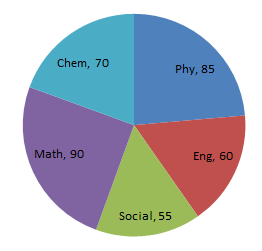Question 31

The difference of marks between Physics and Chemistry is same as that between

Instructions

For the following questions answer them individually

Question 32

Two equal vessels are filled with the mixture of water and milk in the ratio of 3:4 and 5:3 respectively. If the mixtures are poured into a third vessel, the ratio of water and milk in the third vessel will be

Question 33

Three bells ring simultaneously at 11 a.m. They ring at regular intervals of 20 minutes, 30 minutes, 40 minutes respectively. The time when all the three ring together next is

Question 34

The ratio of the volumes of water and glycerine in 240cc of a mixture is 1:3. The quantity of water (in cc that should be added to the mixture so that the new ratio of the volume of water and glycerine becomes 2:3 is

Question 35

The average mathematics marks of two Sections A and B of Class IX in the annual examination is 74. The average marks of Section A is 77.5 and that of Section B is 70. The ratio of the number of students of Section A and B

Question 36

If 78 is divided into three parts which are in the ratio 1 : $$\frac{1}{3}$$ : $$\frac{1}{6}$$, the middle part is?

Question 37

A and B are partners in a business. A contributes 1/4 of the capital for 15 months and B received 2/3 of the profit. Find for how long B's money was used?

Question 38

If the ratio of areas of two equilateral triangles is 9 : 16, then the ratio of their corresponding sides is

Question 39

If x : y = 4 : 5, then (3x + y) : (5x + 3y) =

Question 40

3 + $$\frac{1}{\sqrt{3}} + \frac{1}{3 + \sqrt{3}} + \frac{1}{\sqrt{3} -3}$$

Question 41

If the arcs of a same length in two circles subtend angles of $$60°$$ and $$75°$$ at their centres, the ratio of their radii is

Question 42

Rs. 700 is divided among A, B, C in such a way that the ratio of the amounts of A and B is 2 : 3 and that of B and C is 4 : 5. Find the amounts in Rs. each received, in order A, B, C.

Question 43

The ratio of monthly incomes of A, B is 6 : 5 and their monthly expenditures are in the ratio 4 : 3. If each of them saves Rs. 400 per month, find the sum of their monthly incomes.

Question 44

15 litres of a mixture contains alcohol and water in the ratio 1 : 4. If 3 litres of Water is mixed in it, the percentage of alcohol in the new mixturewill be

Question 45

ABC is a right angled triangle, B being the right angle. Mid-points of BC and AC are respectively B’ and A’. The ratio of the area of the quadrilateral AA’ B’B to the area of the triangle ABC is

Question 46

The third proportional to $$(\frac{x}{y}+\frac{y}{x}) and \sqrt{x^{2}+y^{2}}$$ is

Question 47

The value of 152(sin 30°+2cos^{2} 45°+3 sin 30°+4cos^{2}45°+.....+17sin30°+18cos^{2}45°) is

Question 48

If the perimeters of a rectangle and a square are equal and the ratio of two adjacent sides of the rectangle is 1 : 2 then the ratio of area of the rectangle and that of the square is

Question 49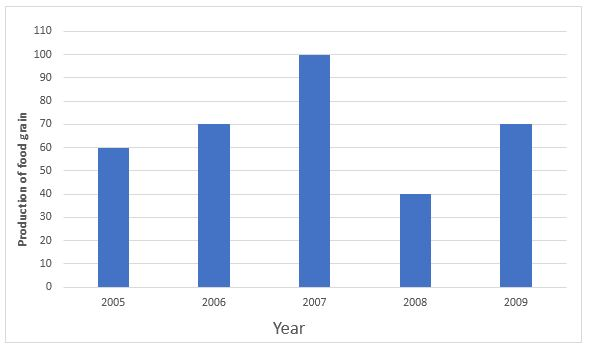Study the above bar graph showing the production of food grains (in million tons). What is the ratio between the maximum production and the minimum production during the given period ?
Graph should be drawn

Question 50

Let BE and CF be the two medians of a $$\triangle$$ ABC and G be their intersection. Also let EF cut AG at O. Then AO : OG is

Question 51

If Rs. 1000 is divided between A and B in the ratio 3:2, then A will receive